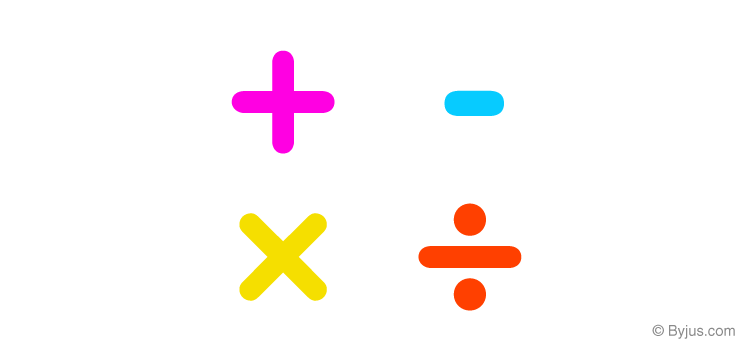# Binary Operation

The basic operations of mathematics- addition, subtraction, division and multiplication are performed on two operands. Even when we try to add three numbers, we add two of them and then add the third number to the result of the two numbers. Thus, the basic mathematical operations are performed on two numbers and are known as binary operations (the word binary means two). In this section, we will discuss binary operations performed on a set.## What is Binary Operation?

We take the set of numbers on which the binary operations are performed as X. The operations (addition, subtraction, division, multiplication, etc.) can be generalised as a binary operation is performed on two elements (say a and b) from set X. The result of the operation on and b is another element from the same set X.

Thus, the binary operation can be defined as an operation * which is performed on a set A. The function is given by *: A * A → A. So the operation * performed on operands a and b is denoted by a * b.

### Types of Binary Operation

There are four main types of binary operations which are:

The complete details for each operation are available in the linked lessons, and an example question is provided below for better understanding.

### Binary Operations Example Questions

• Let us show that addition is a binary operation on real numbers (R) and natural numbers (N). So if we add two operands which are natural numbers a and b, the result will also be a natural number. The same holds good for real numbers. Hence,

+ : R x R → R is given by (a, b) → a + b

+ : N x N → N is given by (a, b) → a + b

• Let us show that multiplication is a binary operation on real numbers (R) and natural numbers (N). So if we multiply two operands which are natural numbers a and b, the result will also be a natural number. The same holds good for real numbers. Hence,

x: R x R → R is given by (a, b) → a x b

x: N x N → N is given by (a, b) → a x b

• Let us show that subtraction is a binary operation on real numbers (R). So if we subtract two operands which are real numbers a and b, the result will also be a real number. The same does not hold good for natural numbers. It is because if we take two natural numbers, 3 and 4 as a and b, then 3 – 4 = -1, which is not a natural number. Hence,

– : R x R → R is given by (a, b)→ a – b

Similarly, the division cannot be defined on real numbers. This is because / : R x R → R is given by (a, b)→ aa/b. Now if we take b as 0 here, a/b is not defined.